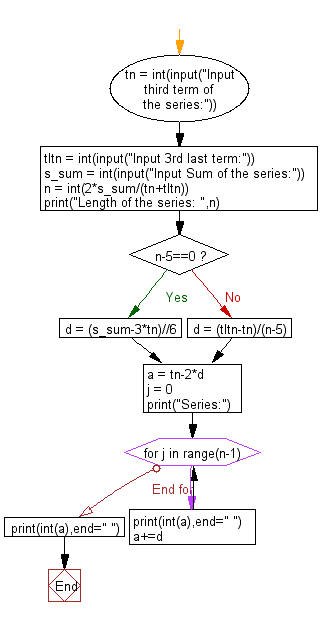﻿ Python: Print the length of the series and the series from the given 3rd term, 3rd last term and the sum of a series - w3resource# Python: Print the length of the series and the series from the given 3rd term, 3rd last term and the sum of a series

## Python Basic - 1: Exercise-28 with Solution

Write a Python program to print the length of the series and the series from the given 3rd term, 3rd last term and the sum of a series.

```Let X and Y denote the third and the third last term of the arithmetic progression respectively
i.e. X=a+2d and Y=a+(n−3)d where a, d and n are what you would expect them to be.
Note that we are given X and Y

Now, we are also given the sum of the n terms i.e. S=n2[2a+(n−1)d]

⇒S=n2[(a+2d)+(a+(n−3)d)]

⇒S=n2[X+Y]

⇒n=2SX+Y

Having computed n, we can plug back it's value in the expression for Y.
This will give us 2 equations in 2 unknowns (a and d) which we can solve to determine the remaining variables.

X=a+2d and Y=a+(2SX+Y−3)d
Reference: https://bit.ly/2N2VM9f
```

Sample Data:
Input third term of the series: 3
Input 3rd last term: 3
Input Sum of the series: 15
Length of the series: 5
Series:
1 2 3 4 5

Sample Solution:

Python Code:

``````tn = int(input("Input third term of the series:"))
tltn = int(input("Input 3rd last term:"))
s_sum = int(input("Sum of the series:"))
n = int(2*s_sum/(tn+tltn))
print("Length of the series: ",n)

if n-5==0:
d = (s_sum-3*tn)//6
else:
d = (tltn-tn)/(n-5)

a = tn-2*d
j = 0
print("Series:")
for j in range(n-1):
print(int(a),end=" ")
a+=d
print(int(a),end=" ")
``````

Sample Output:

```Input third term of the series: 3
Input 3rd last term: 6
Sum of the series: 36
Length of the series:  8
Series:
1 2 3 4 5 6 7 8
```

More Sample Output:

```Input third term of the series: 3
Input 3rd last term: 3
Sum of the series: 15
Length of the series:  5
Series:
1 2 3 4 5
```

Flowchart:## Visualize Python code execution:

The following tool visualize what the computer is doing step-by-step as it executes the said program:

Python Code Editor:

Have another way to solve this solution? Contribute your code (and comments) through Disqus.

What is the difficulty level of this exercise?

Test your Programming skills with w3resource's quiz.

﻿

## Python: Tips of the Day

Iterating over dictionaries using 'for' loops:

I am a bit puzzled by the following code: d = {'x': 1, 'y': 2, 'z': 3} for key in d: print key, 'corresponds to', d[key] What I don't understand is the key portion. How does Python recognize ...

key is just a variable name.

```for key in d:
```

For Python 3.x:

```for key, value in d.items():
```

For Python 2.x:

```for key, value in d.iteritems():
```

To test for yourself, change the word key to poop.

In Python 3.x, iteritems() was replaced with simply items(), which returns a set-like view backed by the dict, like iteritems() but even better. This is also available in 2.7 as viewitems().

The operation items() will work for both 2 and 3, but in 2 it will return a list of the dictionary's (key, value) pairs, which will not reflect changes to the dict that happen after the items() call. If you want the 2.x behavior in 3.x, you can call list(d.items()).

Ref: https://bit.ly/37dm0Qo